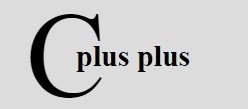# C++ Data Encapsulation

« Previous Tutorial Next Tutorial »

Encapsulation refers to wrapping up of data and associated functions into one single unit. Encapsulation is an Object Oriented Programming (OOP) concept that binds together the data and the functions that manipulate the data, and that keeps both safe from the outside the interference and misuse. Data encapsulation led to the important OOP concept of data hiding. Here is an example:

```class JAVAENCAPSULATIONEXAMPLE
{
public:
double getVol(void)
{
return len * bre * hei;
}
private:
double len;
double bre;
double hei;
};```

The variables len, bre, and hei are private. This means that they can be accessed only by other members of the JAVAENCAPSULATIONEXAMPLE class, and not by any other part of your program. This is one way encapsulation is achieved.

### Access Levels in C++

Here are the access levels with details provided, that helps you in showing/hiding the data from outside the world:

• public - all objects can access
• protected - access becomes limited to members of the same class or descendants only
• private - access becomes limited to members of the same class only

## C++ Data Encapsulation Example

Any C++ program where you implement a class with public and private members is an example of data encapsulation and data abstraction. Consider the following example:

```/* C++ Data Encapsulation - C++ Data Encapsulation Example
* This program demonstrates the concept
* of data encapsulation in C++ */

#include<iostream.h>
#include<conio.h>

class JAVAENCAPSULATIONEXAMPLE
{
public:
JAVAENCAPSULATIONEXAMPLE(int temp=0)
{
tot=temp;
}
{
tot = tot+num;
}
int get_tot()
{
};
private:
int tot;
};

void main()
{
clrscr();
JAVAENCAPSULATIONEXAMPLE a;
cout<<"Sum = "<<a.get_tot() <<endl;
getch();
}```

Here is the sample output of the above C++ program:

Let's take one more example, also demonstrating data encapsulation in C++ practically:

```/* C++ Data Encapsulation - Example Program */

#include<iostream.h>
#include<stdlib.h>
#include<conio.h>
class CALC
{
public:
CALC(int i=0)
{
res=i;
}
{
res=num1+num2;
}
void subnumber(int num1, int num2)
{
res=num1-num2;
}
void mulnumber(int num1, int num2)
{
res=num1*num2;
}
void divnumber(int num1, int num2)
{
res=num1/num2;
}
int getresult()
{
return res;
}
private:
// hidden from outside the world
int res;
};
void main()
{
clrscr();
CALC cob;
int a, b;
char ch;
cout<<"Enter two number: ";
cin>>a>>b;
cout<<"Enter the operator(+,-,/,*): ";
cin>>ch;
switch(ch)
{
case '+':
cout<<"Summation = "<<cob.getresult()<<"\n";
break;
case '-':
cob.subnumber(a, b);
cout<<"Subtraction = "<<cob.getresult()<<"\n";
break;
case '*':
cob.mulnumber(a, b);
cout<<"Multiplication = "<<cob.getresult()<<"\n";
break;
case '/':
cob.divnumber(a, b);
cout<<"Division = "<<cob.getresult()<<"\n";
break;
default:
cout<<"Wrong operator...Exiting..Press a key..";
getch();
exit(1);
}
getch();
}```

Below are some sample runs of the above C++ program:

C++ Online Test

« Previous Tutorial Next Tutorial »